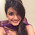The Best Books for Time Series Analysis

 Follow @ProbabilityPuzIf you are looking to learn time series analysis, the following are some of the best books in time series analysis.

Introductory Time Series with R (Use R!)This is good book to get one started on time series. A nice aspect of this book is that it has examples in R and some of the data is part of standard R packages which makes good introductory material for learning the R language too. That said this is not exactly a graduate level book, and some of the data links in the book may not be valid.

EconometricsA great book if you are in an economics stream or want to get into it. The nice thing in the book is it tries to bring out a oneness in all the methods used. Econ majors need to be up-to speed on the grounding mathematics for time series analysis to use this book. Outside of those prerequisites, this is one of the best books on econometrics and time series analysis.

Pattern Recognition and Machine Learning (Information Science and Statistics)This is excellent book to own for scientists and engineers wanting to use time series methods in machine learning, forecasting and regression. Great charts and fairly readable text.

Time Series: Theory and Methods (Springer Series in Statistics)A good book which covers a lot of theoretical aspects but with little practical applications covered. It comes with software so it doesn't really support open source alternatives like R/Python. This is all about a rigorous treatment to Time series analysis (as is the case with most Springer Series). Good for graduate students and academics.

Time Series AnalysisAn ideal book for graduate students and it is fairly comprehensive. Lots of essential approaches are covered in this text. Typical ones include Bayesian approaches, Spectral Analysis and the newer vector auto regression. Strongly recommended for graduate students. The book does not cover real world data sets and applications in enough detail.

Time Series Analysis and Forecasting by Example (Wiley Series in Probability and Statistics)
A good book to get an introduction to time series analysis. It stresses more on the signal processing aspects too like auto regressive models. A drawback is the book requires software and does not use open source, likewise there aren't answers to the questions posted. All said a good book to own but do not forget the caveats.

1.Amzing Post Dear………keep posting!!!
Personalized photo wall clock

The Best Books to Learn Probability

If you are looking to buy some books in probability here are some of the best books to learn the art of Probability

The Probability Tutoring Book: An Intuitive Course for Engineers and Scientists (and Everyone Else!)
A good book for graduate level classes: has some practice problems in them which is a good thing. But that doesn't make this book any less of buy for the beginner.

An Introduction to Probability Theory and Its Applications, Vol. 1, 3rd Edition
This is a two volume book and the first volume is what will likely interest a beginner because it covers discrete probability. The book tends to treat probability as a theory on its own

Discovering Statistics Using R
This is a good book if you are new to statistics & probability while simultaneously getting started with a programming language. The book supports R and is written in a casual humorous way making it an easy read. Great for beginners. Some of the data on the companion website could be missing.

Fifty Challenging Probl…

The Best Books for Linear Algebra

The following are some good books to own in the area of Linear Algebra.

Linear Algebra (2nd Edition)
This is the gold standard for linear algebra at an undergraduate level. This book has been around for quite sometime a great book to own.

Linear Algebra: A Modern Introduction
Good book if you want to learn more on the subject of linear algebra however typos in the text could be a problem.

Linear Algebra (Dover Books on Mathematics)
An excellent book to own if you are looking to get into, or want to understand linear algebra. Please keep in mind that you need to have some basic mathematical background before you can use this book.

Linear Algebra Done Right (Undergraduate Texts in Mathematics)
A great book that exposes the method of proof as it used in Linear Algebra. This book is not for the beginner though. You do need some prior knowledge of the basics at least. It would be a good add-on to an existing course you are doing in Linear Algebra.

Linear Algebra, 4th Edition
This is good book …